ZhouYongyi's Personal Blog# UIUC《文本检索与搜索引擎》课程学习笔记（六）—— 排名训练与推荐系统

## Guiding Questions

Develop your answers to the following guiding questions while completing the readings and working on assignments throughout the week.

• What’s the basic idea of learning to rank?
• How can logistic regression be used to combine multiple features for improving ranking accuracy of a search engine?
• What is content-based information filtering?
• How can we use a linear utility function to evaluate a filtering system? How should we set the coefficients in such a linear utility function?
• How can we extend a retrieval system to perform content-based information filtering?
• What is the exploration-exploitation tradeoff?
• How does the beta-gamma threshold learning algorithm work?
• What is the basic idea of collaborative filtering?
• How does the memory-based collaborative filtering algorithm work?
• What is the “cold start” problem in collaborative filtering?

• C. Zhai and S. Massung. Text Data Management and Analysis: A Practical Introduction to Information Retrieval and Text Mining, ACM Book Series, Morgan & Claypool Publishers, 2016. Chapters 10 – Section 10.4, Chapters 11

## Key Phrases and Concepts

Keep your eyes open for the following key terms or phrases as you complete the readings and interact with the lectures. These topics will help you better understand the content in this module.

• Learning to rank, features, and logistic regression
• Content-based filtering
• Collaborative filtering
• Beta-gamma threshold learning
• Linear utility
• User profile
• Memory-based collaborative filtering
• Cold start

## 一、学习排名（Learning to Rank）

### 1.如何联系多个特征量（How Can We Combine Many Features）

• 一般的思想：
– 给定一个查询-文档对（Q,D），定义多种特征量Xi(Q,D)
– 特征量举例：交叠项的数量、Q和D的BM25算法得分p(Q|D)、D的页面排名p(Q|Di)，其中Di可能是锚文本或者大字体文字(big font text)、URL是否包含’~’ 等
– 假设p(R=1|Q,D)=s(X1(Q,D),…,Xn(Q,D),λ)其中λ是一个参数集
– 将数据输入拟合函数s来训练λ，其中数据为三元组（D,Q,1）(D与Q相关)或者（D,Q,0）(D与Q无关)

### 2.基于回归的方法（Regression-Based Approaches）

$log\frac{P(R=1|Q,D)}{1-P(R=1|Q,D)}=\beta_0+\sum_{i=1}^{n}\beta_iX_i$

$P(R=1|Q,D)=\frac{1}{1+exp(-\beta_0-\sum_{i=1}^n\beta_iX_i)}$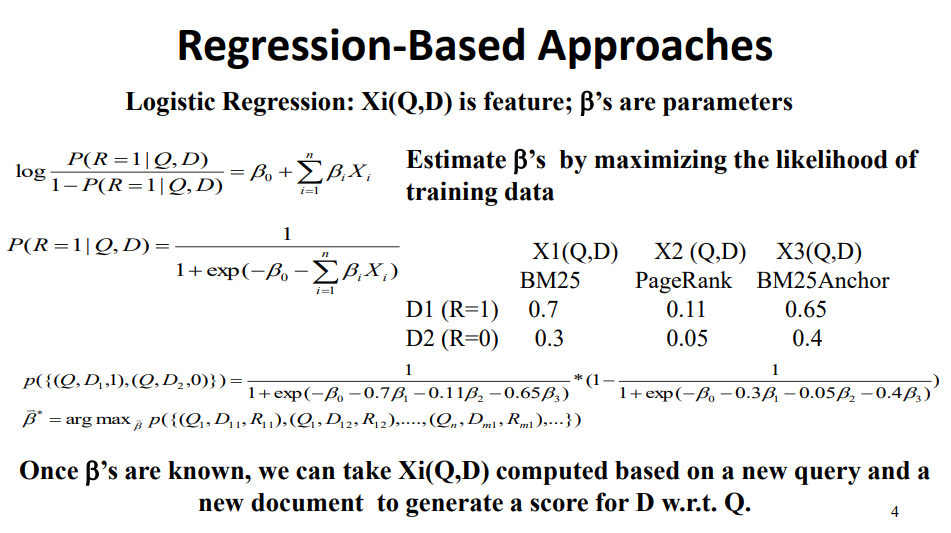• 尝试直接对检索方法进行优化（例如，MAP，nDCG）
– 作为一个优化问题而言更为困难
– 现在有很多新的解决方案
• 能够被应用于搜索问题之外的排名问题
– 推荐系统
– 计算广告
– 概要
– …

### 3.总结（Summary）

• 几十年前，机器学习就已经被用于文本检索（例如Rocchio feedback）
• 现今对于机器学习的应用一般有如下特点：
– 有海量的训练集可用
– 需要联系多种特征量
– 需要健壮的排名算法（比如剔除垃圾信息）
• 现代的网络搜索引擎都用了多种机器学习技术来将多种特征量联系起来，进而优化排名算法
• 学习排名仍然是一个活跃的研究主题

## 二、网络搜索的未来（Future of Web Search）

### 1.下一代的搜索引擎（Next Generation Search Engines）

• 更多的特殊化/定制化（垂直搜索引擎）
– 特定的用户群（社区引擎，例如Citeseer）
– 个性化（更好的了解用户）
– 特殊的类型/领域（更好地理解文档）
• 随着时间推移进行学习（进化）
• 搜索积分，导航，以及推荐/过滤（丰富的信息管理）
• 对搜索之外的任务提供支持（例如购物）
• 很多技术革新的机会

### 2.数据-用户-服务三角（Data-User-Service Triangle, DUS）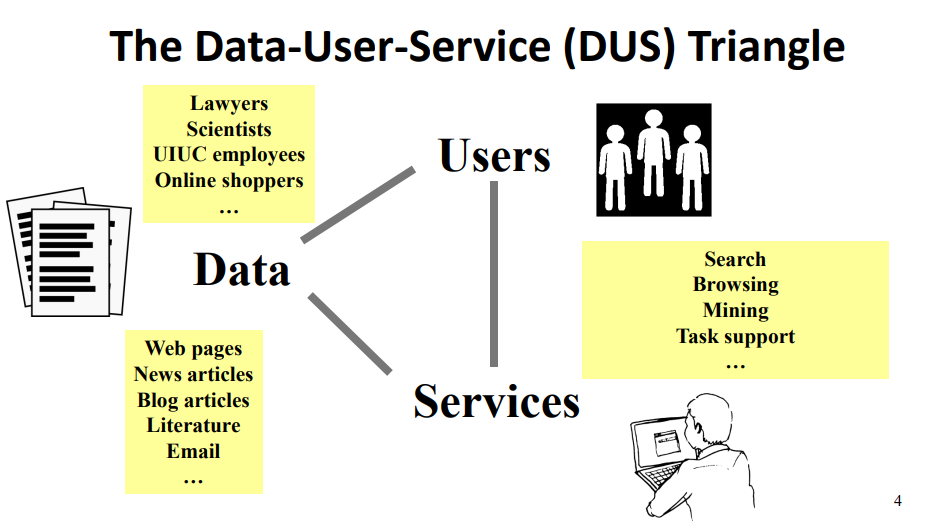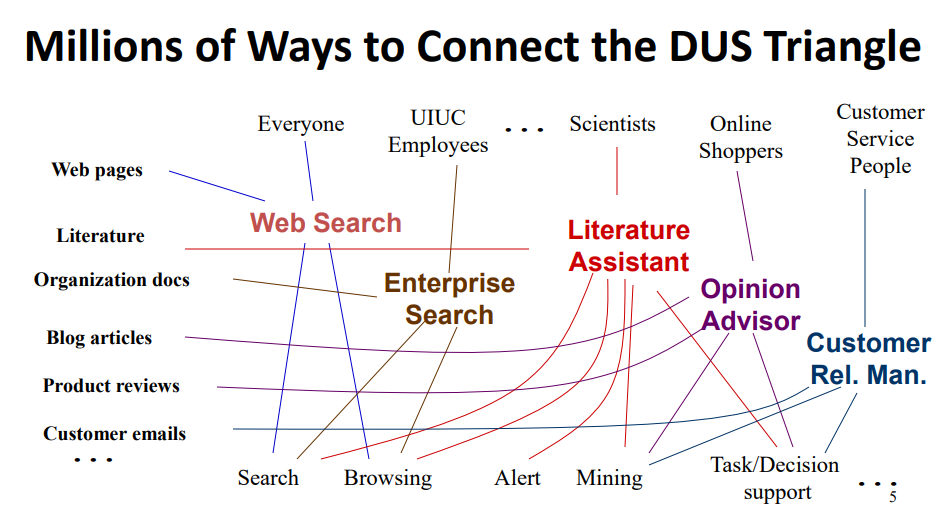### 3.未来的智能信息系统（Future Intelligent Information Systems）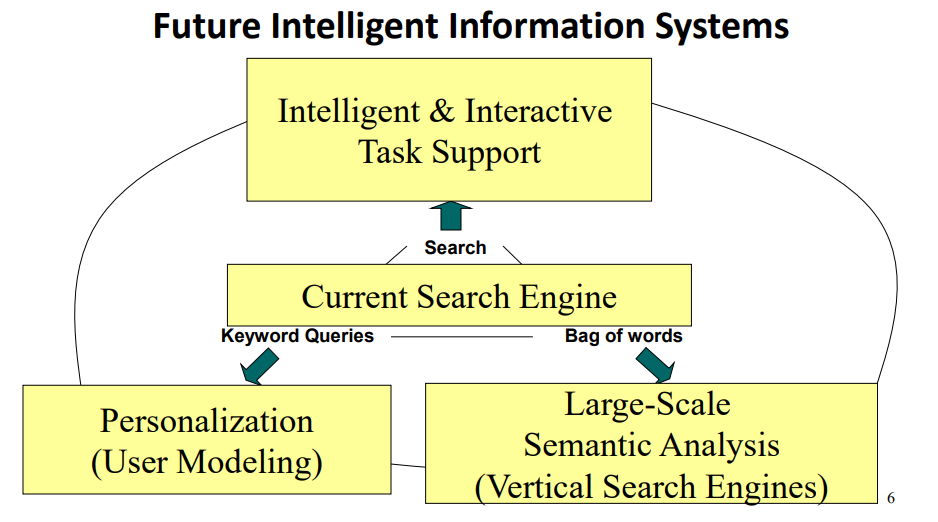## 三、推荐系统：基于内容的过滤（Recommender Systems: Content-Based Filtering）

### 1.文本获取的两种模式：拉和推（Two Modes of Text Access: Pull vs. Push）

• 拉模式（Pull，搜索引擎）
– 用户进行初始化
– 需要临时信息
• 推模式（Push, 推荐系统）
– 系统进行初始化
– 需要有稳定的信息源或者系统需要对用户有足够的了解

### 2.推荐≈过滤系统（Recommender ≈ Filtering System）

• 稳定且长期的兴趣，动态的信息源
• 系统需要在文档到达之后迅速做出决策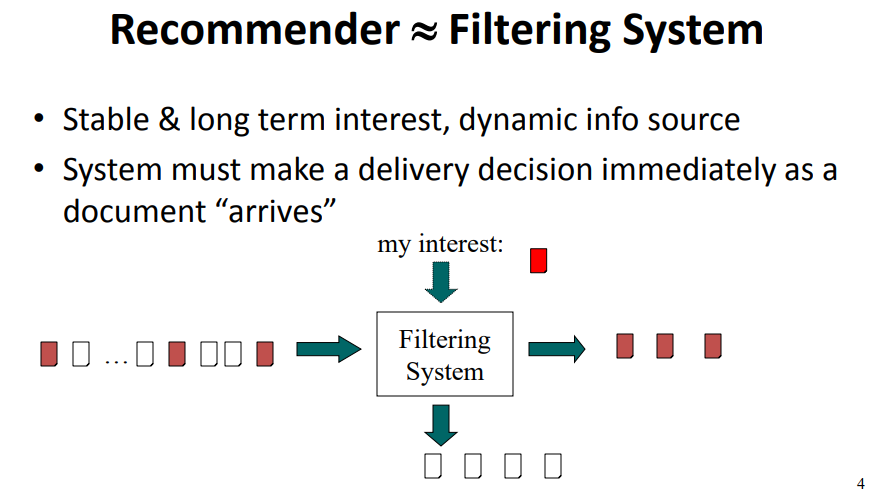### 3.基本的过滤问题：用户U会喜欢物品X吗？（Basic Filtering Question: Will User U Like Item X?）

• 两种不同的方式来回答这个问题
– 先看看用户U喜欢什么东西，然后看看X和这些是否相似
物品相似  => 基于内容过滤（content-based filtering）
– 先看看谁喜欢物品X，再看看U和他们是否相似
用户相似  => 协同过滤（collaborative filtering）
• 这两种方式能够被同时使用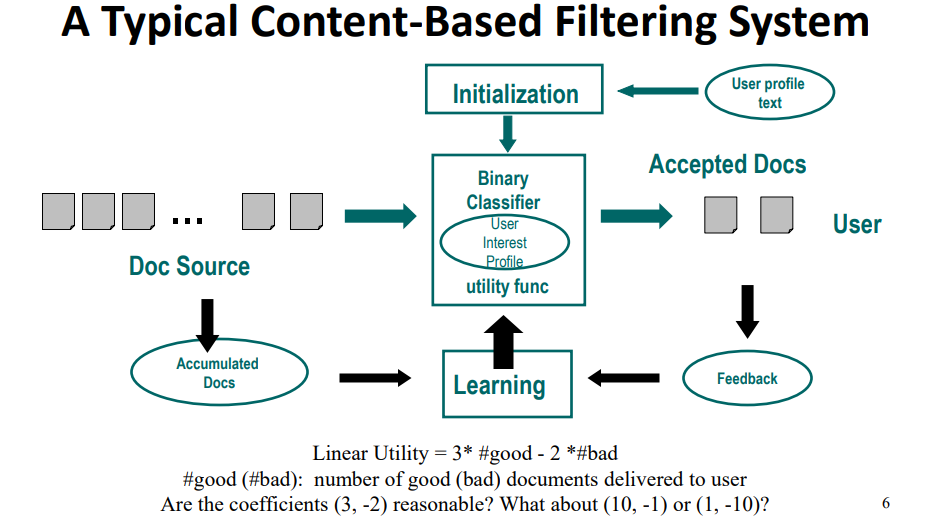### 4.内容过滤中的三个基本问题（Three Basic Problems in Content-Based Filtering）

• 做出过滤决策（二分分类）
– 文档文本，个人资料 -> yes/no
• 初始化
– 只通过个人资料或者很少的例子来初始化过滤器
• 通过如下内容进行训练
– 特定的相关性判断（只针对yes文档）
– 累积文档
• 都需要尝试效果最大化

### 5.为信息过滤器扩展一个检索系统（Extend a Retrival System for Information Filtering）

• “重用reuse”检索技术来进行文档评分
• 使用一个分数阈值来进行过滤决策
• 尝试使用反馈来优化评分系统
• 使用新的方法来进行阈值设定与学习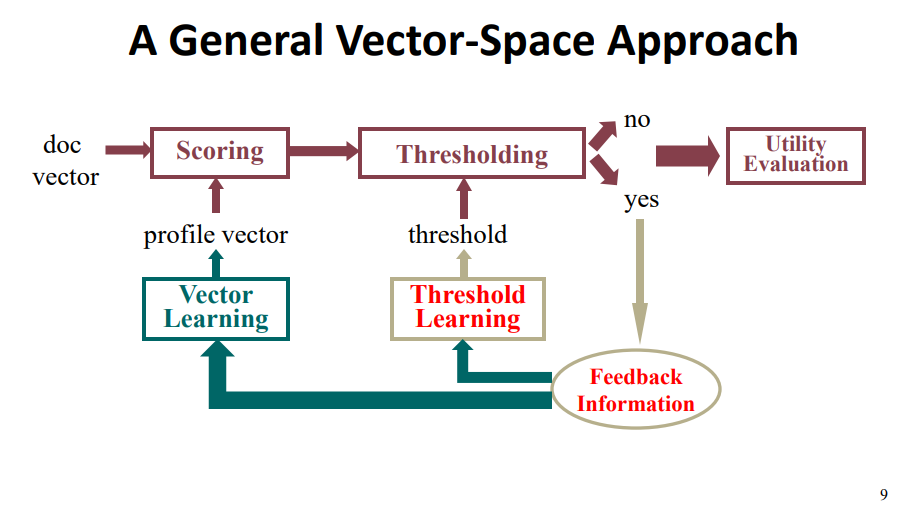• 审查数据（只能用已知的文档进行判断）
• 很少/没有 标记好的数据
• 勘探与开发，即，以什么程度来从被过滤掉的物品中挖掘出潜在的用户可能感兴趣的东西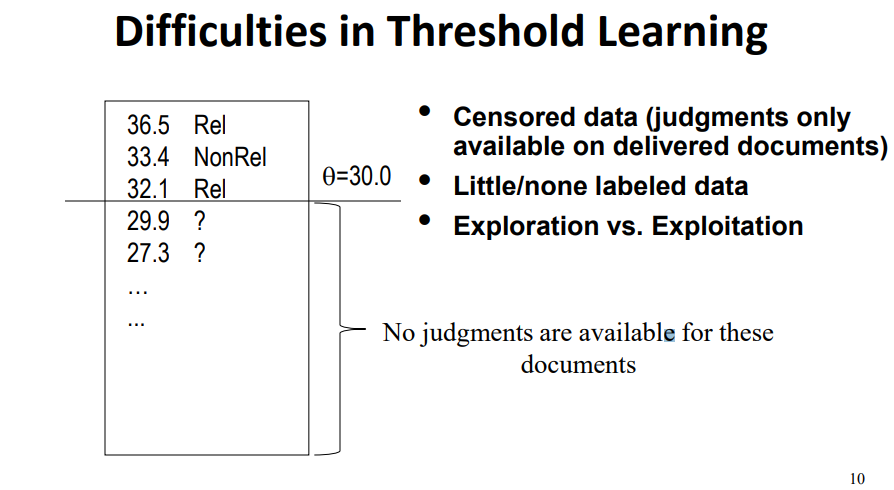### 6.经验优化（Empirical Utility Optimization）

• 基本思想
– 计算不同阈值下对训练集的作用效果
– 选择效果最好的那个阈值
• 难点：训练集的偏差（biased）
– 我们只能得到该阈值的最理想情况下的效果
– 被过滤掉的物品中会不会有潜在的对用户有用的东西？
• 解决方案
– 启发式地调整（降低）阈值

### 7.Beta-Gamma阈值学习（Beta-Gamma Threshhold Learning）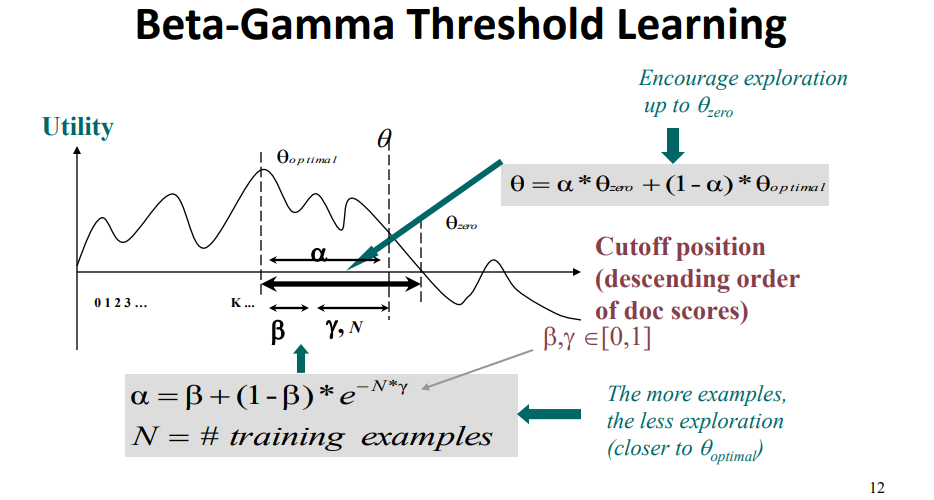• 优点
– 明确地界定了勘探-开发（exploration-exploitation）的权衡问题，即，以什么程度来挖掘用户的潜在兴趣
– 任意性效果（使用适当较低的边界）
– 经验优化
• 缺点
– 纯粹是启发式的
– 零效率下界太过于保守

### 8.总结（Summary）

• 两种推荐/过滤策略
– 基于内容过滤（物品相似）
– 协同过滤（用户相似）
• 基于内容的推荐系统能够在一个搜索引擎的基础上构建出来
– 添加阈值机制
– 添加自适应的学习算法

## 四、推荐系统：协同过滤（Recommender System: Collaborative Filtering）

### 1.什么是协同过滤（What is Collaborative Filtering, CF）

• 通过判断一个用户与其他用户的相似度来进行过滤决策
• 通过相似用户的特点来判别个体的喜好
• 基本思想:
– 给定一个用户u，找出其相似用户{u1,…,um}
– 基于相似用户的特点，来预测用户u的喜好
– 用户的相似度可以通过他们喜欢的物品集合来进行判断

### 2.协同过滤的假设（CF: Assumptions）

• 有相同兴趣的用户会有相似的喜好
• 有相似喜好的用户可能会有相同的兴趣
• 例如
– 兴趣是信息检索 => 喜欢SIGIR期刊
– 喜欢SIGIR期刊 => 对信息检索有兴趣
• 有海量的用户行为数据可以使用（如果没有的话，会变成一个冷启动问题）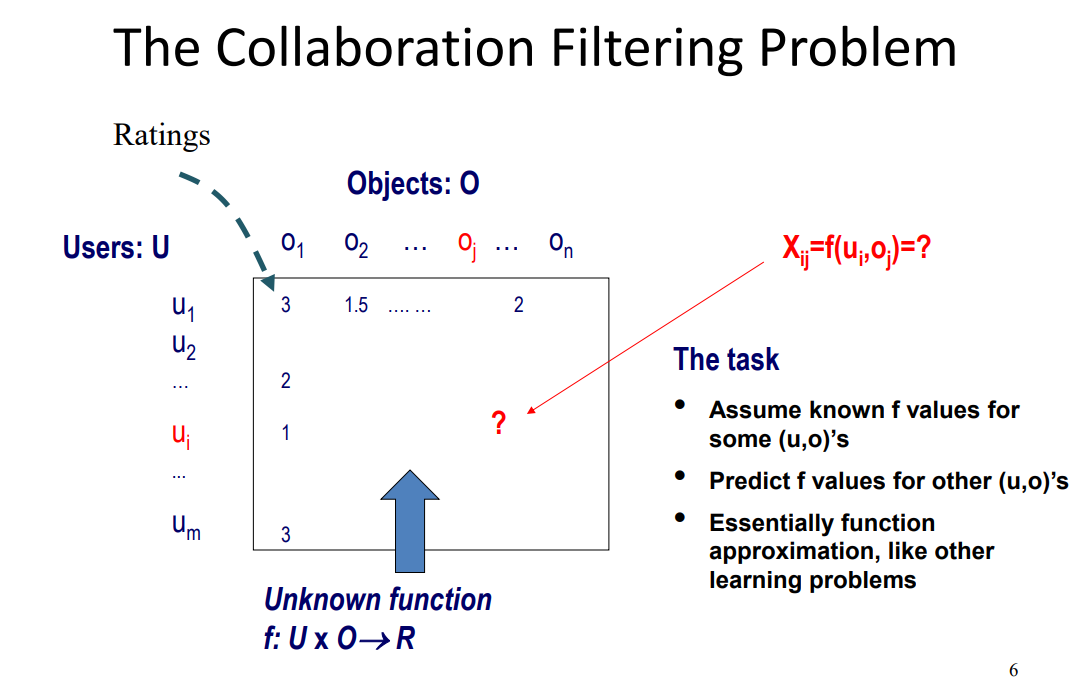### 3.基于内存的方式（Memory-based Approaches）

• 一般思想：
– X_ij:用户u_i对物品o_j的评分
– n_i: 用户u_i对所有物品的平均评分
– 标准化评分：V_ij=X_ij-n_i
– 预测用户u_a对于物品o_j的评分

$\hat{v}_{aj}=k\sum_{i=1}^mw(a,i)v_ij$

$\hat x_{aj}=\hat y_{aj}+n_a$

$k=\frac{1}{\sum_{i=1}^mw(a,i)}$

• 在计算w(a,i)的时候有所不同——基于用户u_a和u_i的距离/相似度

### 4.用户相似度计算（User Simiarity Measures）

• Pearson相关系数（基于所有评分物品的总和）

$w_p(a,i)=\frac{\sum_j(X_{aj}-n_a)(X_{ij}-n_i)}{\sqrt{\sum_j(X_{aj}-n_a)^2\sum_j(X_{ij}-n_i)^2}}$

• 余弦方法

$w_c(a,i)=\frac{\sum_{j=1}^nX_{aj}X_{ij}}{\sqrt{\sum_{j=1}^nX_{aj}^2\sum_{j=1}^nX_{ij}^2}}$

• 还有很多其他方法……

### 5.改进用户相似度算法（Improving User Similarity Measures）

• 处理丢失的值：设定一个缺省评分（例如，平均评分）
• 相对用户频率（Inverse User Frequency, IUF）:和IDF一样

### 6.推荐系统总结（Summary）

• 过滤/推荐很容易
– 用户的期望值很低
– 有推荐总比没推荐要好
• 过滤很难
– 需要作出二分决策，尽管也可以使用排名算法
– 数据解析（限制了反馈信息）
– 冷启动问题（关于一个用户的信息一开始是很少的）
• 基于内容的过滤 和 协同过滤 和 Hybrid
• 推荐能够与搜索结合起来：Push Pull
• 现在提出了很多进阶算法，它们使用了更多文本信息以及进阶的机器学习
Tags:

Insert math as
$${}$$